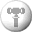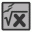# CalculatorX 1.2: Free Download

Download Locations
 Size : 1.28 MBwww.qwerks.com

CalculatorX 1.2 Description
 The enhanced expression calculator. [ Read More ]

Related DownloadsGraphe Easy A feature-rich yet easy-to-use 2d-graph plotting Windows software.GraphCalc An all-in-one Windows graphing calculator. A must for any high school math student.Function Grapher Plot 2D, 2.5D and 3D graphs of math equations and tables.Math Calculator Abilities of expression calculating, solving equation, finding extremum, derivative calculating and integral calculating.MultyGraphiX Calculation software designed to plot 2D and 3D functions.

 POPULAR LINKS